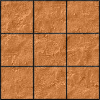#### You may also like### Pyramids

What are the missing numbers in the pyramids?### Paving the Way

A man paved a square courtyard and then decided that it was too small. He took up the tiles, bought 100 more and used them to pave another square courtyard. How many tiles did he use altogether?### Chess

What would be the smallest number of moves needed to move a Knight from a chess set from one corner to the opposite corner of a 99 by 99 square board?

# The Simple Life

##### Age 11 to 14 Challenge Level:

When Colin simplified the expressions below, he was surprised to find that they all gave the same solution! Try it for yourself.

$$3(x+6y) + 2(x-5y)$$$$4(2x-y) - 3(x-4y)$$$$-2(5x-y) + 3(5x+2y)$$

Here is a set of five expressions: $$(x + y) \quad (x + 2y) \quad (x - 2y) \quad (x + 4y) \quad (2x + 3y)$$

Choose any pair of expressions and add together multiples of each (like Colin did).

Can you find a way to get an answer of $5x+8y$ in each case?

Warning... you will have to multiply the expressions by fractions in some cases.

If you're struggling to get started... take a look below to see how Charlie and Alison thought about the problem when combining multiples of $(x+2y)$ and $(2x+3y)$.

Charlie's trial and improvement approach:

Charlie chose a value for $a$ and worked out the value of $b$ that gave $5x$.
He then kept adjusting the values of $a$ and $b$ until he also got $8y$:

 $a$ $b$ $a(x+2y) + b(2x+3y)$ $5$ $0$ $5x+10y$ $4$ $\frac {1}{2}$ $5x+9\frac {1}{2}y$ $3$ 1 $5x+9y$ $2$ $\frac {3}{2}$ $5x+8\frac {1}{2}y$ $1$ 2 $5x+8y$

Alison's algebraic approach:

Alison multiplied out the brackets:\eqalign{a(x+2y)+b(2x+3y)&=5x+8y \\ \Rightarrow \begin{cases}ax+2bx &= 5x\\ 2ay+3by &= 8y \end{cases} \\ \Rightarrow \begin{cases} a+2b &= 5 \\ 2a+3b &= 8 \end{cases} } \\ \Rightarrow a=1 \quad \text{and} \quad b=2

With thanks to Colin Foster who introduced us to this problem.